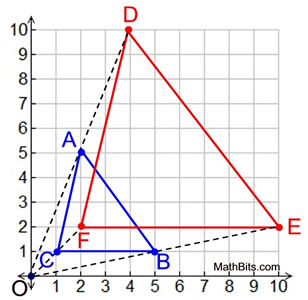Practice - Grid Dilations Terms of Use    Contact Person: Donna Roberts1.
a) Find the image of the point (-3,3) under a dilation in the origin of scale factor ½.
Choose:
 (1.5,1.5) (3,3) (-1.5,1.5) (-3,-3)

b)
Find the image of the point (-3,3) under a dilation in the origin of scale factor 1.5.
Choose:
 (-5,5) (4.5,3) (5,-5) (-4.5,4.5)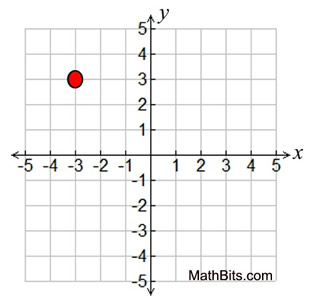2.
Under a dilation centered in the origin, rectangle A becomes rectangle B.
What is the scale factor for this dilation?

Choose:
 2 2½ 3 3½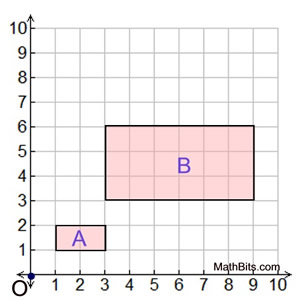3.
ΔABC has the vertices shown. Find the coordinates of its image, ΔA'B'C', after a dilation of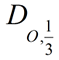Choose:
 A'(18,18), B'(27,0), C'(9,-9) A'(3,18), B'(9,0), C'(1,-9) A'(2,2), B'(3,0), C'(1,-1) A'(3,3), B'(4.5,0), C'(1.5,-1.5)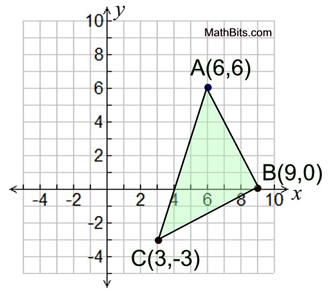4.
ΔABC has A(-2,-2), B(-1,2) and
C
(2,1). After a dilation centered at the origin, ΔA'B'C' has A'(-4,-4),
B'(-2,4) and C'(4,2). What is the scale factor of the dilation?

Choose:
 -2 2 ½ -½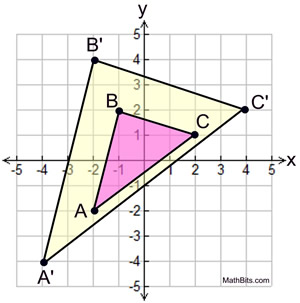5.
Pentagon ABCDE is shown. What will be the coordinates of the image of D after a dilation of scale factor ½ centered in the origin?
Choose:
 (-1, -½) (-1,½) (0,-½) (0,½)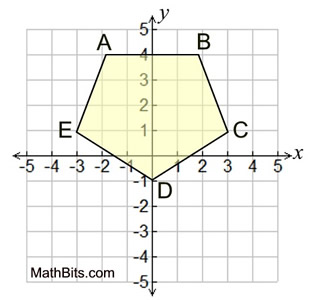6.
Rectangle ABCD is shown. What are the coordinates of the image of point B after a dilation of scale factor 2 centered in point A?

Choose:
 (2,8) (2,4) (4,4) (7,4)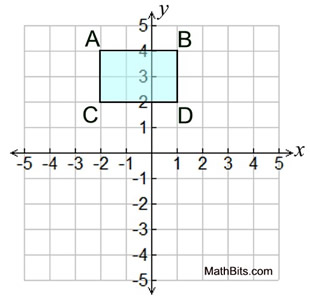7.
ΔFUN is shown at the right. Find the coordinates of its image ΔF'U'N' under a dilation of 3 centered in the origin.

Choose:
 F(-8,2), U(6,8), N(0,-6) F(-2,½), U(1½,2), N(0,-1½) F(-12,3), U(9,12), N(3,-9) F(-12,3), U(9,12), N(0,-9)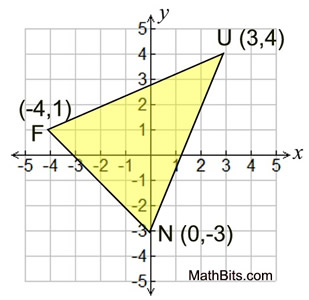8.

Arrow B is the result of one, or more, transformation on arrow A,
as shown at the right.

Which choice describes the transformation(s)?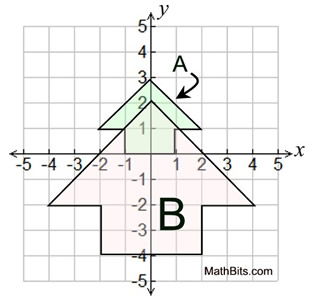Choose:
 dilation of A, scale factor 2, center origin. dilation of A, scale factor 2, center origin, followed by a translation of 4 units down. dilation of A, scale factor 3, center origin, followed by a translation of 2 units down. dilation of A, scale factor 3, center origin, followed by a translation of 4 units down.

9.
ΔABC is graphed as shown. What are the coordinates of the vertices of the image ΔA'B'C' after a dilation of scale factor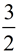centered in the origin?
Choose:
 A(2,9), B(3,0), C(-3,3) A(4½,9), B(3,0), C(-3,3) A(4½,9), B(3,1½), C(-3,3) A(9,18), B(6,0), C(-6,6)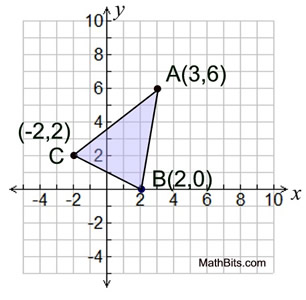10.
A dilation centered in the origin, O, creates ΔDEF as the image of ΔABC.
a) What is the scale factor for this dilation?

Choose:
 ¼ ½ 1½ 2

b)
The length of OC is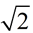. What is the length of OF ?

Choose: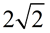2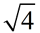4